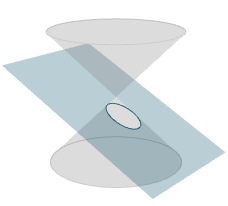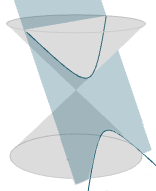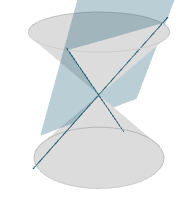Search IntMath
Close

# Conic sections interactive applet

By Murray Bourne, 25 May 2016

I recently updated the conic sections interactive applet, part of the Plane Analytic Geometry section.

The applet now uses a different back-end meaning that it's now mobile-friendly and somewhat more convincing.

Using the applet you can explore the conic shapes that occur when you slice a double cone by a plane at various angles and positions.

You will get either a circle, ellipse, parabola or hyperbola. Such curves are called conic sections, since they are the result of slicing a cone with a plane.

## Example output

When the plane's angle is less than the cone's side angle, we get an ellipse, as follows:Conic section - Ellipse

If the angle of the plane is greater than the angle of the sides of the cone, the result is a hyperbola:Conic section - Hyperbola

At various extremeties, you get a degenerate conic, which is either a:

• Point (e.g. when the plane passes through the point joining the two cones)
• Intersecting lines (e.g. when the plane is parallel to the axis of the two cones, and passes through the origin)

Here's an example of the latter case:Degenerate hyperbola (2 straight lines)

## Developing the interactive applet

I developed the applet using the brilliant Three.js library by MrDoob.

Constructing each of the conic section curves should have been a straightforward process of finding the intersection between the plane (at various angles) and the cone(s), then looping around the resulting curve at small intervals and joining the points.

However, each conic type threw up its own challenges. For example, I could draw the hyperbolas on the x-y (horizontal) plane, then rotate them at the correct angle and then move them into position, but the other curves didn't play nicely with that approach and I had to try all sorts of different ways.

The "fun" issue is that eah situation was multi-variable. That is, by being able to move the plane left and right, and up and down, and at different angles, a lot of testing was necessary to make sure it all worked as intended.

### The math involved

There's always a lot of mathematical thinking involved in creating these interactive applets, as there is for creating any interactive visual app, especially games.

For the current conic sections applet, I needed to use and calculate:

1. 3D vectors and angles
3. Intersection between a cone and a plane
4. Trigonometric ratios (sin, tan)
5. Finding square roots
6. Ellipse formula
7. Parabola formula
8. Hyperbola formula
9. Straight line formula
10. Slopes of lines in 2D and 3D
11. Rotation angles in 2D and 3D
12. Modelling (finding a function to fit given data, e.g. for the degenerate hyperbola cases)

The link again: Conic Sections Summary Interactive

See the 1 Comment below.

### Comment Preview

HTML: You can use simple tags like <b>, <a href="...">, etc.

To enter math, you can can either:

1. Use simple calculator-like input in the following format (surround your math in backticks, or qq on tablet or phone):
a^2 = sqrt(b^2 + c^2)
(See more on ASCIIMath syntax); or
2. Use simple LaTeX in the following format. Surround your math with $$ and $$.
$$\int g dx = \sqrt{\frac{a}{b}}$$
(This is standard simple LaTeX.)

NOTE: You can mix both types of math entry in your comment.